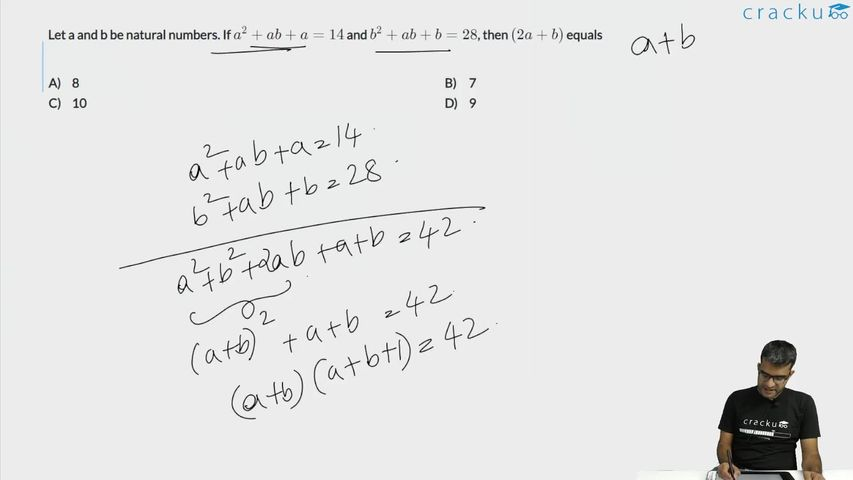Question 55

# Let a and b be natural numbers. If $$a^2 + ab + a = 14$$ and $$b^2 + ab + b = 28$$, then $$(2a + b)$$ equals

Solution

a(a + b + 1) = 14 ...... (1)

b(a + b + 1) = 28 ...... (2)

$$\frac{a}{b}=\frac{1}{2}$$

b = 2a

Substituting in (1), we get

a(3a + 1) = 14

$$3a^2+a-14=0$$

$$3a^2-6a+7a-14=0$$

$$3a\left(a-2\right)+7\left(a-2\right)=0$$

Given, a and b are natural numbers.

Therefore, a = 2 and b = 4

2a + b = 2(2) + 4 = 8

### View Video Solution• All Quant CAT Formulas and shortcuts PDF
• 30+ CAT previous papers with solutions PDF